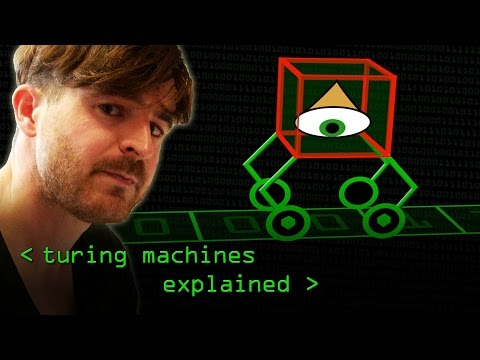## OISC#

There is a programming language that has only one function. It is known as Single Instruction Programming Language or One Instruction Set Compiler (OISC) or Ultimate Reduced Instruction Set Computer (URISC).

Before diving deep, what is “Instruction Set” ?

An instruction set, or instruction set architecture (ISA), is the part of the computer architecture related to programming, including the native data types, instructions, registers, addressing modes, memory architecture, interrupt and exception handling, and external I/O. An ISA includes a specification of the set of opcodes (machine language) and the native commands implemented by a particular processor.

Coming back, we also need to have an understanding of the Turing machine and Turing completeness. There is an amazing computerphile video regarding this:The OISC that I am going to talk about is also Turing complete, but the best part is unlike the Turing machine it doesn’t need to have an infinite memory model. Hence, it is equivalent to a real computer than a Turing machine. For any instruction to be Turing Complete (or in simple words be able to compute most complex computations) it needs to have some sort of conditional branching. You can imagine this to be something like

``````if <condition>
goto <branch>
``````

Now, these machines can be categorized into 3 types:

1. Bit manipulating machines
2. Transport triggered machines
3. Arithmetic-based machines

`mov` is one such instruction. The `mov` instruction copies the data item referred to by its second operand (i.e. register contents, memory contents, or a constant value) into the location referred to by its first operand (i.e. a register or memory).

``````mov a, b
``````
``````//it is equivalent to
*a = *b;
``````

This `mov` instruction is Turing complete and comes under 2. Transport Triggered Machine. There is an amazing repo that showcases the fact that any C program can be compiled into a program written only using `mov` instructions.Arithmetic-based Turing-complete machines use an arithmetic operation and a conditional jump. Let’s take an example of one such instruction. The `subleq` which stands for Subtract and branch if less than or equal to zero

`````` subleq a, b, c   ; Mem[b] = Mem[b] - Mem[a]
; if (Mem[b] ≤ 0) goto c
``````

So, you can see there is a basic arithmetic operation and one conditional branching, (we already mentioned that it is required to be Turing complete). There is a syntactic sugar for the same which goes like this

`````` subleq a, b   ; Mem[b] = Mem[b] - Mem[a]
; goto next instruction
``````

Here we basically drop the `c` which means we aren’t branching anywhere rather moving on to the next instruction. Now the big question. Is this instruction enough? Well as it turns out. Yes, it is.

Let’s look at some programs using this instruction:

``````; initially *z = 0
subleq a, z
subleq z, b
subleq z, z
``````

let’s break this down

``````//initally
*z = 0;

//line 1
*z = *z - *a // since *z=0, this line boils down to
*z = -*a

//line 2
*b = *b - *z // *z = -*a, this line boils down to
*b = *b - (- *a)
*b = *b + *a

//line 3
*z = *z - *z
*z = 0 //we started with *z=0 and ended with *z=0
``````

What we see is `*b = *b + *a` i.e. this 3 line instruction performs addition.

`The reason we use (*) before a, b, z is because those represent memory addresses and *a represents the value in that particular memory block`

`The reason the last line is important is because we have to ensure we do not end up changing the values that we are not dealing with, here we were just dealing with a and b. z was initially 0 and finally should also be 0. In other words, there shouldn't be any side effects`

Not just addition other instructions can also be implemented with `subleq` instruction as well.

``````; assignment b = a
; initially *z=0
subleq b, b
subleq a, z
subleq z, b
subleq z, z
``````

You can work these out and you will come to the conclusion that it ends up assigning the value of a to b. Or you can apply 200IQ and notice that this is exactly the same as the prev one, only a new instruction `subleq b, b` was added to the beginning, we know the other 3 lines implement this `*b = *b + *a` and the first line is basically `*b=0` , hence what we finally get is `*b= 0+*a` i.e. `*b=*a`.

``````; jump to branch c
subleq z, z, c
``````

This is very basic, it will just jump to the branch `c`.

The interesting thing is this programming language with this one instruction is equally powerful to any other programming language like C++, Java .etc. It may not be very efficient and easy to write but in the end, all of these comes under the class of Turing complete. Yet there are some interesting benefits in employing a one instruction computer. For example, hardware-level functionality is simplified when implemented around a single instruction. This greatly simplifies the underlying implementation, as the same functional element is repeatedly used to form the processor core. Another advantage is that since all the instructions are the same, the instruction decoder circuitry and complexity can be eliminated.

Why should we study OISC ?

OISC architectures provide an excellent paradigm for implementing traditional von Neumann computers using non-traditional materials. Simply put, by massive scaling of simple, single instruction elements, a practical computer can be built.Ref: